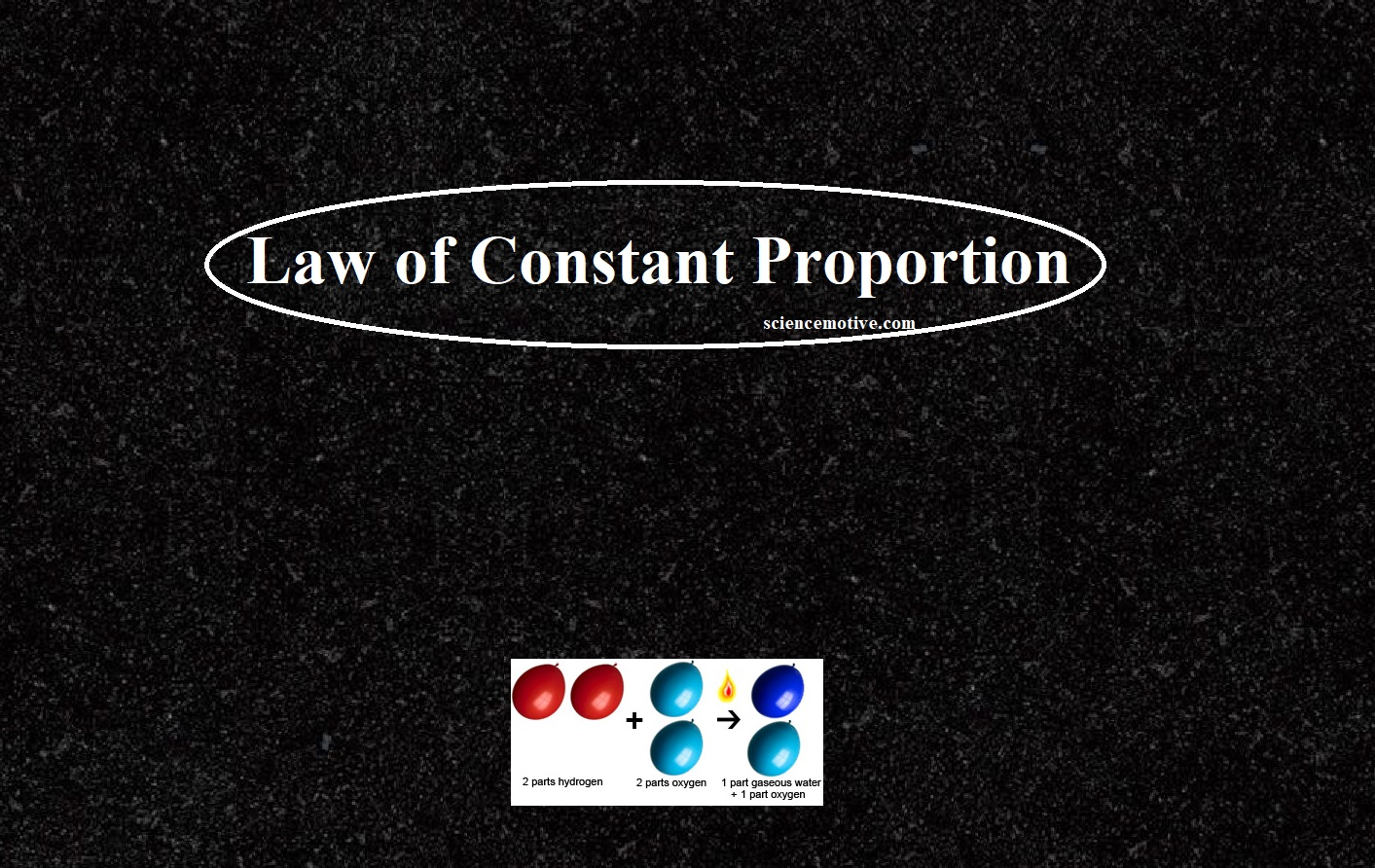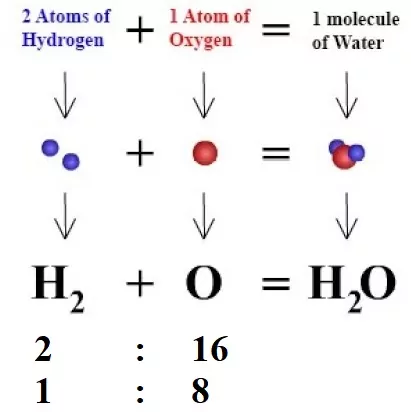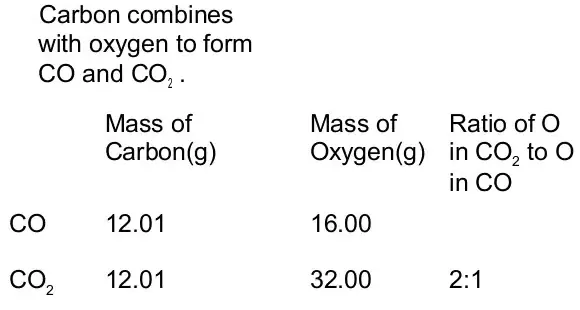# Law of Constant Proportion – Explanation, Examples and Numerical Problems# Law of Constant Proportion

Law of Constant Proportion

The combination of elements to form compounds is governed by the following five basic laws:
Law of Conservation of Mass
Law of Constant Proportion
Law of Multiple Proportions
Gay Lussac’s Law of Gaseous Volumes

In Chemistry, the Law of Definite Proportion is sometimes called Proust’s law or the law of constant composition. According to this law, a chemical compound always contains the same elements combine together in a fixed ratio by mass. This implies that any pure sample of a compound, no matter the source, will always consist of the same elements that are present in the same ratio by mass.
For example, pure water obtained from different sources such as a river, a well, a spring, the sea, etc., always contains hydrogen and oxygen together in the ratio of 1:8 by mass.Similarly, carbon dioxide (CO2) can be obtained by different methods such as,
* Burning of carbon of fuel
* Respiration
* Heating of limestone
* Applying dilute HCl to marble pieces
Each sample of CO2 contains carbon and oxygen in a 3:8 ratio.The law of constant proportion is not valid in all cases. Elements with a stable isotope mixture often form a non-stoichiometric product. The role of certain elements in the crystal structure is replaced by their isotopes which induces the crystal’s internal composition to vary.
Significance of the law of constant proportions:
it was of great use in the late 18th century when chemical compounds did not have any proper definition. The law of definite proportions also contributed to the development of Dalton’s atomic theory.

Numerical problems on Law of Constant Proportion

Que 1. When 1.375 g of cupric oxide is reduced on heating in a current of hydrogen, the weight of copper remaining 1.098 g. In another experiment, 1.179 g of copper is dissolved in nitric acid and the resulting copper nitrate is converted into cupric oxide by ignition. The weight of cupric oxide formed is 1.476 g. Show that these results illustrate the law of constant proportion.
Ans 1. First case:
Copper oxide taken = 1.375 g
Copper left = 1.098 g
Oxygen present = 1.357 g – 1.098 = 0.277 g
Hence, % of O in CuO = (0.277 x 100)/(1.357) = 20.14
Second case :
Copper taken = 1.179 g
Copper oxide formed = 1.476 g
Oxygen present = 1.476 g – 1.179 = 0.297 g
Hence, % of O in CuO = (0.297 x 100)/(1.476) = 20.12
Hence, percentage of oxygen is same in both of the above cases, so the is proved.

Que 2. Two gaseous samples were analyzed. One contained 1.2g of carbon and 3.2 g of oxygen. The other contained 27.3 % carbon and 72.7% oxygen. The above data is in accordance with, the law of definite proportion?
Ans 2. The total mass of both gases = Mass of Carbon + Mass of Oxygen
= 1.2 + 3.2 = 4.6 g
% of C in the 1st sample = (1.2/4.6) × 100 = 27.3%
Which is the same as in the second sample. Hence the law of definite proportion is obeyed.

Law of Constant Proportions